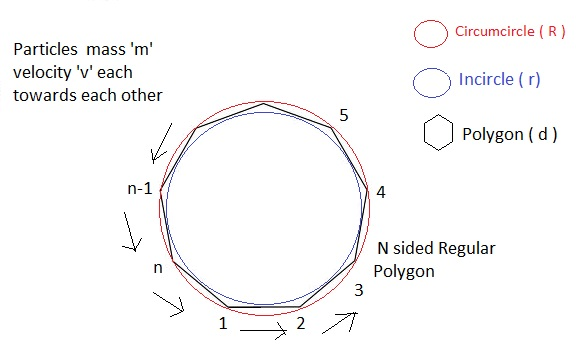# Geometry + Mechanics = GeonicsLet us consider an Regular n-sided Polygon of side length d , Initially n identical particles each of mass m are placed rest at the vertices of the polygon. Then at time t=0 they start moving with constant speed ${ v }_{ o }$ each such that they always moves in direction towards the adjacent particle. Let the total time taken by particles when they meets is T .

If circum-radius of the Regular polygon is R and it's in-radius is r .

If the value of
$\frac { r }{ R } \quad =\quad \sqrt { 1-\quad (\cfrac { x }{ y } \times \frac { d }{ { v }_{ o }T } ) }$.

Then Find value of $1729\quad \times \quad \cfrac { x }{ y } \quad$

Details and assumptions

$\bullet$ x and y are positive co-prime integers.